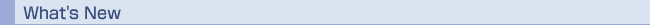超ロバスト計算原理プロジェクト　最適化・制御セミナーのご案内 　日時：平成18年9月29日（金）　15:00〜17:15 　場所：工学部6号館3階セミナー室C 【講演 I 】(15:00-16:00) 講演者：Dr. Gongyun Zhao （National University of Singapore） タイトル：Representing the space of linear programs as a Grassmannian 概要 We represent the space of linear programs as the space of projection matrices. Projection matrices of the same dimension and rankcomprise a Grassmannian, which has rich geometric andalgebraic structures. An ordinary differential equation on the space of projection matrices defines a path for each projection matrix associated with a linear programming instance and the path leads to a projection matrix associated with an optimal basis of the instance. In this way, any point （projection matrix） in the Grassmannian is connected to a stationary point of the differential equation. We will present some basic properties of the stationary points, in particular, the characteristics of eigenvalues and eigenvectors. We will show that there are only a finite number of stable points. Thus, the Grassmannian can be partitioned into a finite number of attraction regions, each associated with a stable point. The structures of the attraction regions will be important for applications which will be discussed at the end of this paper. 【講演 II 】(16:15-17:15) 講演者：Dr. Renato D. C. Monteiro （Georgia Institute of Technology） タイトル：First-order primal-dual algorithms for cone programming 　（Joint work with G. Lan, Z. Lu, A. Nemirovski） 概要 In this talk, we discuss first-order algorithms for solving cone programming problems. The algorithm consists of applying well-known first-order methods due to Nesterov and Nemirovski to suitable convex smooth and non-smooth reformulations of the cone programming problem. We compare the different variants obtained in this manner among themselves. We also compare the performance of the most Efficient one of these variants agaisnt the low-rank method of Burer and et al.'s on a set of randomly generated semidefinite programming problems. （連絡先：COE特任教授　土谷　隆　内線：28608）